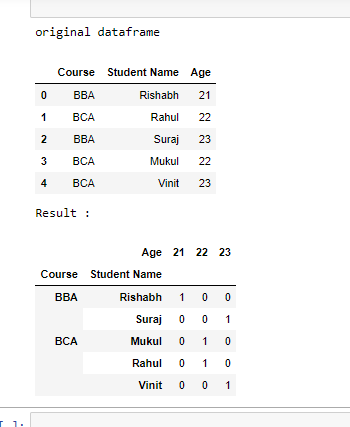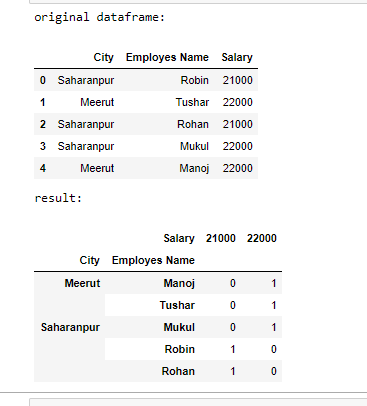Related Articles

# Pandas – Groupby value counts on the DataFrame

• Last Updated : 15 Mar, 2021

Prerequisites: Pandas

Pandas can be employed to count the frequency of each value in the data frame separately. Let’s see how to Groupby values count on the pandas dataframe. To count Groupby values in the pandas dataframe we are going to use groupby() size() and unstack() method.

### Functions Used:

• groupby(): groupby() function is used to split the data into groups based on some criteria. Pandas objects can be split on any of their axes. The abstract definition of grouping is to provide a mapping of labels to group names

Syntax:

DataFrame.groupby(by=None, axis=0, level=None, as_index=True, sort=True, group_keys=True, squeeze=False, **kwargs)

Parameters :

• by : mapping, function, str, or iterable
• axis : int, default 0
• level : If the axis is a MultiIndex (hierarchical), group by a particular level or levels
• as_index : For aggregated output, return object with group labels as the index. Only relevant for DataFrame input. as_index=False is effectively “SQL-style” grouped output
• sort : Sort group keys. Get better performance by turning this off. Note this does not influence the order of observations within each group. groupby preserves the order of rows within each group.
• group_keys : When calling apply, add group keys to index to identify pieces
• squeeze : Reduce the dimensionality of the return type if possible, otherwise return a consistent type

Returns : GroupBy object

• size(): size method is used to get the integer representing the number of elements in object. The size method is return the number of rows times number of columns if DataFrame.

Syntax:

Dataframe.size()

• unstack(): unstack method works with the MultiIndex objects in DataFrame, producing a reshaped DataFrame with a new inner-most level of column labels.

Syntax:

Dataframe.unstack()

### Approach

• Import module
• Create data frame
• Count value of occurrences of each value
• Print resultant data frame

Example1:

## Python

 `# import pandas``import` `pandas as pd`` ` `# create dataframe``df ``=` `pd.DataFrame({``    ``'Course'``: [``'BBA'``, ``'BCA'``, ``'BBA'``, ``'BCA'``, ``'BCA'``],``    ``'Student Name'``: [``'Rishabh'``, ``'Rahul'``, ``'Suraj'``, ``'Mukul'``, ``'Vinit'``],``    ``'Age'``: [``21``, ``22``, ``23``, ``22``, ``23``]})`` ` ` ` `# print original dataframe``print``(``"original dataframe"``)``display(df)`` ` `# counts Groupby value``df ``=` `df.groupby([``'Course'``, ``'Student Name'``, ``'Age'``]).size().unstack(fill_value``=``0``)`` ` `# print dataframe``print``(``"Result :"``)``display(df)`

Output:Example 2:

## Python

 `# import pandas``import` `pandas as pd`` ` `# create dataframe``df ``=` `pd.DataFrame({``    ``'City'``: [``'Saharanpur'``, ``'Meerut'``, ``'Saharanpur'``, ``'Saharanpur'``, ``'Meerut'``],``    ``'Employes Name'``: [``'Robin'``, ``'Tushar'``, ``'Rohan'``, ``'Mukul'``, ``'Manoj'``],``    ``'Salary'``: [``21000``, ``22000``, ``21000``, ``22000``, ``22000``]})`` ` ` ` `# print original dataframe``print``(``"original dataframe: "``)``display(df)`` ` `# counts Groupby value``df ``=` `df.groupby([``'City'``, ``'Employes Name'``, ``'Salary'``]``                ``).size().unstack(fill_value``=``0``)`` ` `# print dataframe``print``(``"result: "``)``display(df)`

Output:Attention geek! Strengthen your foundations with the Python Programming Foundation Course and learn the basics.

To begin with, your interview preparations Enhance your Data Structures concepts with the Python DS Course. And to begin with your Machine Learning Journey, join the Machine Learning – Basic Level Course

My Personal Notes arrow_drop_up